Understanding Chemical Bonding

Understanding Chemical Bonding

Introduction

Key Terms

• Orbital
• Principle quantum number
• Pauli exclusion principle
• Spin quantum number
• Valence electron
• Ionic bond
• Cation
• Anion
• Covalent bond
• Valence shell
• Octet rule
• Lewis structures
• Electronegativity
• Dipole moment

Objectives

• Review broadly the quantum physics foundation for the study of organic chemistry
• Represent atoms (elements) according to their electron configuration
• Recognize ionic and covalent bonds between atoms and some of their basic characteristics
• Use Lewis structures to represent molecules (combinations of elements)

Resources

Organic chemistry is the study of carbon compounds and their interactions. Organic compounds (or chemicals) are the foundation of life, and from this fact arose the name "organic." But as you probably know, an organic compound is not always conducive to life: a wide variety of man-made organic compounds are toxic. Nevertheless, organic chemistry involves the study of even these toxic compounds, in addition to the carbon-based molecules that are central to biology. Before we begin looking at different types of carbon compounds, their nomenclature, and how they interact, we must first consider (lightly) the characteristics of atoms that govern how molecules are formed and how they interact.

Quantum Mechanics: A Foundation for Chemistry

Chemistry deals primarily with the interactions of elements (or atoms) and the larger compounds they form by combining with each other. The periodic table organizes the known elements according to their properties-specifically, by their number of protons and arrangement of electrons. Each element is composed of some number of protons (the atom's atomic number, which is sometimes written as Z) and some number of neutrons combined in the nucleus. Surrounding the nucleus is some number of electrons (the same as the number of protons for an individual neutral atom). Early models of the atom view the structure as a "solar system," with the nucleus being a star orbited by planets (electrons). Protons (positively charged) attract electrons (negatively charged) by way of the electric force.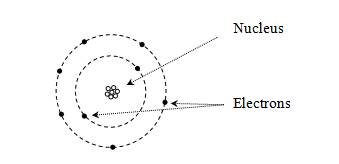But this model, although intuitively appealing, has numerous problems. According to quantum theory, electrons do not act like little planets orbiting a sun-like nucleus; instead, they act more like waves, and their position around the nucleus is indefinite. Thus, they are modeled as "clouds," and the position of each electron is expressed in terms of a probability that it is in a particular region. (This probability is the square of the electron's wave function, which is an important topic in quantum mechanics.) Furthermore, the energy associated with an electron (which we might view as its "energy of motion") can only be certain discrete values, and these energy levels are associated with the shape of the electron's probability distribution (or wave function). Melding the quantum mechanical concept of a wave function with the early "planetary" view of electrons, a particular wave function is often called an orbital. Consider a simple case of a single proton and a single electron (a hydrogen atom); the lowest-energy orbital is a spherical probability distribution like the one shown below, where the nucleus is centered at the origin. Note that the electron is more likely to be closer to the center than farther out.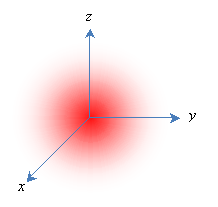Because orbitals extend to infinity (for instance, the electron in a hydrogen atom has a finite-although very small-probability of being some extremely large distance from the proton, even though it spends most of its time very close), orbitals are often depicted as solid shapes. These shapes represent the region in which the electron exists most of the time-an arbitrary figure around 90%. We would then represent the above orbital as follows.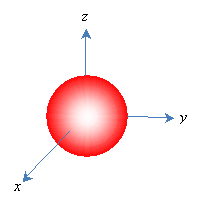Classifying Orbitals

When an atom has multiple electrons, these electrons are distributed in different orbitals, and this distribution is crucial to understanding how atoms interact. First, orbitals are classified by the principle quantum number, a value that determines the orbital's energy level. This number can be any positive integer, with the lowest-energy orbital having a value of 1. Because physical systems tend to minimize their associated energy, electrons tend to fill lower-energy orbitals before higher-energy ones.

Second, orbitals are loosely classified by their shapes, with s representing spherical orbitals and p representing "dumbbell-shaped" orbitals. The lowest-energy orbital is the 1s orbital and looks like the one sketched above. The p orbitals are similar to the sketch below, with one p orbital associated with each axis (px, py, and pz, with px shown below). Again, the nucleus is located at the origin.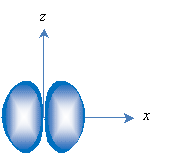The principle quantum number 1 only has a single orbital associated with it (1s), but succeeding principle quantum numbers have a an s orbital and three p orbitals. The p orbitals have a higher energy than the s orbitals with the same principle quantum number.

Finally, we must note that the Pauli exclusion principle dictates that each orbital can only contain two electrons, which are differentiated by their spin quantum numbers of either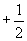or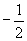. The number of electrons in a particular orbital (either one or two) is typically written as a superscript after the s or p. Thus, hydrogen (in its lowest energy state) has an electron configuration 1s1, because it has a single electron in the 1s orbital. Following the periodic table, then, helium has an electron configuration 1s2; lithium, 1s22s1; beryllium, 1s22s2; boron, 1s22s22px1; and so on. Often, the different p orbitals for a given principle quantum number are combined, so that, for example, chlorine is written as 1s22s22p63s23p5. The electrons associated with an atom's largest principle quantum number are often called valence electrons and are typically the main contributors to how an atom interacts with other atoms.

Practice Problem: What is the electron configuration of carbon? What about argon?

Solution: A periodic table is a helpful tool in writing electron configurations. Carbon has an atomic number 6, meaning it has six protons and six electrons. Filling the orbitals from lowest energy to highest energy with these six electrons, we find that carbon has an electron configuration 1s22s22p2. (Note that adding the superscripts yields six.) Argon has an atomic number 18, meaning it has 18 electrons. Its electron configuration is therefore 1s22s22p63s23p6.

Chemical Bonding

Although different elements (atoms) have their own unique properties, the numerous ways in which they combine is what gives organic chemistry (and chemistry in general) its numerous and detailed contours. Chemical bonds are the forces that cause atoms and molecules to attract, and the strongest of these bonds are ionic bonds and covalent bonds.

An ionic bond is the result of electrostatic attraction between two oppositely charged ions (atoms or molecules that have more or fewer electrons than the total number of protons, causing a net positive or negative charge). In some cases, an atom may be able to dissociate from an electron very easily; in such cases (such as elements on the left side of the periodic table), the atom may form a cation (positively charged ion). If the atom easily accepts an additional electron (for instance, elements on the right side of the periodic table, excepting the noble gases), it may form an anion (negatively charged ion). This process is illustrated in the reaction of gaseous (g) sodium and gaseous chlorine, which exchange an electron and then attract one another electrostatically, forming an ionic bond.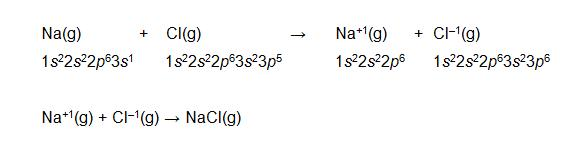Note that in this case, sodium releases its 3s electron to chlorine, which fills its 3p orbital with this electron. The resulting ions attract one another through the electric force.

An ionic bond is characterized by donation and acceptance of an electron (or a number of electrons); a covalent bond, on the other hand, is characterized by sharing of an electron pair. Some elements may not easily dissociate an electron, but their electron configurations are not as stable as they could be. In such cases, sharing of an electron pair with another atom may increase the stability of the electron configurations for one or both of the atoms. But what constitutes a stable electron configuration? If you look carefully at the reaction above that illustrates an ionic bond, you may notice that the electron exchange between sodium and chlorine gives both atoms a full set of valence electrons (or a full valence shell). Likewise, other atoms tend to seek full valence shells, which are characterized by greater stability: this is the octet rule, and we will almost invariably apply this rule.

Consider the following example of a covalent bond formed between two chlorine atoms. Note that we use dots to represent the valence electrons of each atom; such representations are known as Lewis structures.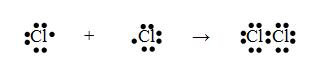Typically, we will show a covalent bond as a single solid line (for example, H-H for a bond between two hydrogen atoms). We will only use dots to represent electrons when they represent a nonbonding (unshared) pair, and we will often omit these dots altogether.

A shared electron pair may not be shared equally-the electrons may spend more time around one atom than around another. This occurs when the electronegativity of one atom exceeds that of the other. (Electronegativity is a property specific to each element, and it can be found in some periodic tables or other compilations of elemental data.) For example, in the molecule HCl, the electrons tend to be associated more with the chlorine atom than with the hydrogen atom (in other words, chlorine has a higher electronegativity). As a result, the molecule, although neutral overall, exhibits a dipole moment (one atom tends to have a net negative charge and the other a net positive charge). In some sense, covalent bonds in these situations are not purely covalent: they have some covalent character and some ionic character. We represent the dipole moment using a special arrow in the direction of the more electronegative element.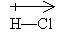Atoms may also have more than one covalent bond between them. Consider nitrogen, for instance: it has five valence electrons (1s22s22p3). One covalent bond between two nitrogen atoms brings each member's electron configuration to 1s22s22p4, and a second covalent bond brings each configuration to 1s22s22p5. A third covalent bond produces a stable, full valence shell: 1s22s22p6. This form, N2, is generally what we think of in common parlance as nitrogen. Its Lewis structure is shown below.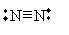And of course, an atom may have covalent bonds with more than one other atom; our primary governing principle in this regard is to maintain the octet rule, except in certain special cases.

Practice Problem: Calcium (Ca) and chlorine (Cl) combine to form CaCl2. How would you describe the bonds that form between these atoms?

Solution: Because calcium is on the left side of the periodic table, it can reach a stable electron configuration (a full valence shell) by dissociating two electrons, whereas chlorine does so by accepting one electron. Thus, CaCl2 is formed through ionic bonds between the chlorine atoms and the calcium atom, giving each a stable electron configuration.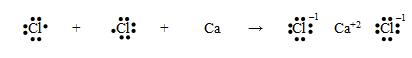Practice Problem: Fluorine (F) combines like chlorine to form F2. Determine if this molecule has a dipole moment.

Solution: Fluorine combines via covalent bonding, as shown in the Lewis structure below.Because both fluorine atoms have the same electronegativity (a property of each element, as mentioned earlier), the electrons are shared equally between each side, so no dipole moment exists.